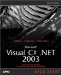Creating Structs

C# also supports structs , which you create with the struct keyword. Structs in C# are like lightweight versions of classes. They're not reference types; they're value types, so when you pass them to methods , they're passed by value. They're like classes in many waysthey support constructors, for example (but not inheritance). They take up fewer resources in memory, so when you've got a small, frequently used class, give some thought to using a struct instead. Here's how you declare a struct :

[  attributes  ] [  modifiers  ] struct  identifier  [:  interfaces  ]  body  [;]

Here are the parts of this statement:

• attributes (Optional) Hold additional declarative information, as we'll see in Chapter 14.

• modifiers (Optional) The allowed modifiers are new , static , virtual , abstract , override , and a valid combination of the four access modifiers.

• identifier The struct name .

• interfaces (Optional) A list that contains the interfaces implemented by the struct, all separated by commas.

• body The struct body that contains member declarations.

Because structs are value types, you can create them without using new (although you can also use new and pass arguments to a struct's constructor).

FOR C++ PROGRAMMERS

In C++, structures are like classes, with only a few minor differences. In C#, structs are value types, not reference types, and cannot support inheritance, initializers, or destructors.

Consider the Complex struct, which holds complex numbers. Complex numbers have both real and imaginary parts (the imaginary part is multiplied by the square root of 1). For example, in the complex number 1 + 2i, the real part is 1 and the imaginary part is 2. In this struct, we'll implement the public fields real and imaginary , as well as a constructor and a method named Magnitude , to return the magnitude of this complex number (which will use the System.Math.Sqrt method to calculate a square root):

struct Complex {   public int real, imaginary;   public Complex(int real, int imaginary)   {     this.real = real;     this.imaginary = imaginary;   }   public double Magnitude()   {     return System.Math.Sqrt(real * real +       imaginary * imaginary);   } }

Now you can create new complex numbers without using new , just as you would for any value type. You can see an example in ch03_06.cs, Listing 3.6, where we create the complex number 3 + 4i (where i is the square root of -1), and display this number's magnitude.

Listing 3.6 Creating a struct (ch03_06.cs)
class ch03_06 {   static void Main()   {  Complex complexNumber;   complexNumber.real = 3;   complexNumber.imaginary = 4;   System.Console.WriteLine("Maginitude: {0}",   complexNumber.Magnitude());  } } struct Complex {   public int real, imaginary;   public Complex(int real, int imaginary)   {     this.real = real;     this.imaginary = imaginary;   }   public double Magnitude()   {     return System.Math.Sqrt(real * real +       imaginary * imaginary);   } }

When you run this example, this is what you see:

C:\>ch03_06 Maginitude: 5Microsoft Visual C#.NET 2003 Kick Start
ISBN: 0672325470
EAN: 2147483647
Year: 2002
Pages: 181

Similar book on Amazon